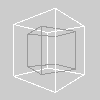Name

applyMatrix()

Examplessize(100, 100, P3D)
noFill()
translate(50, 50, 0)
rotateY(PI/6)
stroke(153)
box(35)
# Set rotation angles
ct = cos(PI/9.0)
st = sin(PI/9.0)
# Matrix for rotation around the Y axis
applyMatrix(  ct,  0.0,  st,  0.0,
0.0, 1.0,  0.0, 0.0,
-st, 0.0,  ct,  0.0,
0.0, 0.0,  0.0, 1.0)
stroke(255)
box(50)
Description Multiplies the current matrix by the one specified through the parameters. This is very slow because it will try to calculate the inverse of the transform, so avoid it whenever possible. The equivalent function in OpenGL is glMultMatrix().
Syntax
applyMatrix(source)
applyMatrix(n00, n01, n02, n10, n11, n12)
applyMatrix(n00, n01, n02, n03, n10, n11, n12, n13, n20, n21, n22, n23, n30, n31, n32, n33)
Parameters
n00 float: numbers which define the 4x4 matrix to be multiplied float: numbers which define the 4x4 matrix to be multiplied float: numbers which define the 4x4 matrix to be multiplied float: numbers which define the 4x4 matrix to be multiplied float: numbers which define the 4x4 matrix to be multiplied float: numbers which define the 4x4 matrix to be multiplied float: numbers which define the 4x4 matrix to be multiplied float: numbers which define the 4x4 matrix to be multiplied float: numbers which define the 4x4 matrix to be multiplied float: numbers which define the 4x4 matrix to be multiplied float: numbers which define the 4x4 matrix to be multiplied float: numbers which define the 4x4 matrix to be multiplied float: numbers which define the 4x4 matrix to be multiplied float: numbers which define the 4x4 matrix to be multiplied float: numbers which define the 4x4 matrix to be multiplied float: numbers which define the 4x4 matrix to be multiplied
Related pushMatrix()
popMatrix()
resetMatrix()
printMatrix()

Updated on Mon Sep 21 15:53:24 2020.

If you see any errors or have comments, please let us know.